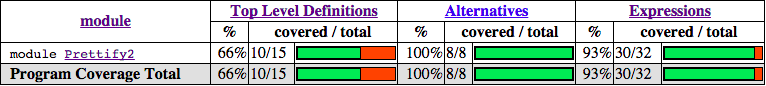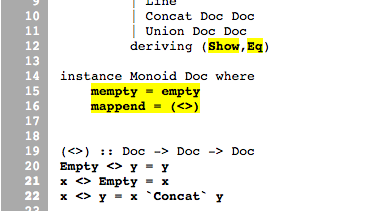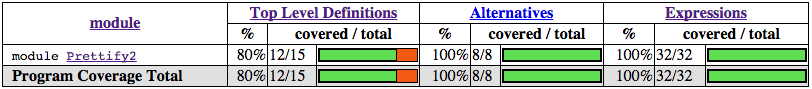# 第十一章：测试和质量保障¶

## QuickCheck: 基于类型的测试¶

```-- file: ch11/QC-basics.hs
import Test.QuickCheck
import Data.List
```

```-- file: ch11/QC-basics.hs
qsort :: Ord a => [a] -> [a]
qsort []     = []
qsort (x:xs) = qsort lhs ++ [x] ++ qsort rhs
where lhs = filter  (< x) xs
rhs = filter (>= x) xs
```

```-- file: ch11/QC-basics.hs
prop_idempotent xs = qsort (qsort xs) == qsort xs
```

[译注，运行之前需要确保自己安装了 QuickCheck 包，译者使用的版本是2.8.1。]

```ghci> prop_idempotent []
True
ghci> prop_idempotent [1,1,1,1]
True
ghci> prop_idempotent [1..100]
True
ghci> prop_idempotent [1,5,2,1,2,0,9]
True
```

[译注：本例子根据最新版本的 QuickCheck 库做了改动。]

```Prelude Test.QuickCheck.Gen Test.QuickCheck.Arbitrary> sample' arbitrary :: IO [Bool]
[False,False,False,True,False,False,True,True,True,True,True]
```

QuickCheck 用这种方法产生测试数据，然后通过 quickCheck 函数把数据传给我们要测试的性质。性质本身的类型决定了它使用哪个数据生成器。 quickCheck 确保对于所有产生的测试数据，性质仍然成立。由于幂等测试对于列表元素类型是多态的，我们需要选择一个特定的类型来产生测试数据， 我们把它作为一个类型约束写在性质上。运行测试的时候，只需调用 quickCheck 函数，并指定我们性质函数的类型即可（否则的话，列表值将会是没什么意思的 () 类型）：

```*Main Test.QuickCheck> :type quickCheck
quickCheck :: Testable prop => prop -> IO ()
*Main Test.QuickCheck> quickCheck (prop_idempotent :: [Integer] -> Bool)
+++ OK, passed 100 tests.
```

### 性质测试¶

```-- file: ch11/QC-basics.hs
import Data.List
prop_minimum xs         = head (qsort xs) == minimum xs
```

```*Main Test.QuickCheck> quickCheck (prop_minimum :: [Integer] -> Bool)
*** Failed! Exception: 'Prelude.head: empty list' (after 1 test):
[]
```

```-- file: ch11/minimum.hs

minimum    :: (Ord a) => [a] -> a
minimum [] =  error "Prelude.minimum: empty list"
minimum xs =  foldl1 min xs
```

```-- file: ch11/QC-basics.hs
prop_minimum' xs         = not (null xs) ==> head (qsort xs) == minimum xs
```

```*Main Test.QuickCheck> quickCheck (prop_minimum' :: [Integer] -> Property)
+++ OK, passed 100 tests.
```

```-- file: ch11/QC-basics.hs
prop_ordered xs = ordered (qsort xs)
where ordered []       = True
ordered [x]      = True
ordered (x:y:xs) = x <= y && ordered (y:xs)

prop_permutation xs = permutation xs (qsort xs)
where permutation xs ys = null (xs \\ ys) && null (ys \\ xs)

prop_maximum xs         =
not (null xs) ==>
last (qsort xs) == maximum xs

prop_append xs ys       =
not (null xs) ==>
not (null ys) ==>
head (qsort (xs ++ ys)) == min (minimum xs) (minimum ys)
```

### 利用模型进行测试¶

```-- file: ch11/QC-basics.hs
prop_sort_model xs      = sort xs == qsort xs
```

## 测试案例学习：美观打印器¶

### 生成测试数据¶

```-- file: ch11/Prettify2.hs

data Doc = Empty
| Char Char
| Text String
| Line
| Concat Doc Doc
| Union Doc Doc
deriving (Show,Eq)
```

QuickCheck 鼓励这样一种测试方式：开发人员指定一些不变量，它们对于任何代码接受的输入都成立。 为了测试美观打印库，我们首先需要一个输入数据源。我们可以利用 QuickCheck 通过 Arbitrary 类型类提供的一套用来生成随机数据的组合子集。 Arbitrary 类型类提供了 arbitrary 函数来给每种类型生成数据，我们可以利用它来给自定义数据类型写数据生成器。

```-- file: ch11/Arbitrary.hs
import Test.QuickCheck.Arbitrary
import Test.QuickCheck.Gen
class Arbitrary a where
arbitrary   :: Gen a
```

```-- file: ch11/Arbitrary.hs
elements :: [a] -> Gen a
choose   :: Random a => (a, a) -> Gen a
oneof    :: [Gen a] -> Gen a
```

elements 函数接受一个列表，返回这个列表的随机值生成器。我们稍后再用 chooseoneof。 有了 elements，我们就可以开始给一些简单的数据类型写生成器了。例如，如果我们给三元逻辑定义了一个新数据类型：

```-- file: ch11/Arbitrary.hs
data Ternary
= Yes
| No
| Unknown
deriving (Eq,Show)
```

```-- file: ch11/Arbitrary.hs
instance Arbitrary Ternary where
arbitrary     = elements [Yes, No, Unknown]
```

```-- file: ch11/Arbitrary2.hs
instance Arbitrary Ternary where
arbitrary     = do
n <- choose (0, 2) :: Gen Int
return \$ case n of
0 -> Yes
1 -> No
_ -> Unknown
```

```-- file: ch11/Arbitrary.hs
instance (Arbitrary a, Arbitrary b) => Arbitrary (a, b) where
arbitrary = do
x <- arbitrary
y <- arbitrary
return (x, y)
```

[译注，作者在此处解释并实现了 CharArbitrary 实例。但由于最新 QuickCheck 已经包含此实例，故此处略去相关内容。]

```-- file: ch11/QC.hs
instance Arbitrary Doc where
arbitrary = do
n <- choose (1,6) :: Gen Int
case n of
1 -> return Empty

2 -> do x <- arbitrary
return (Char x)

3 -> do x <- arbitrary
return (Text x)

4 -> return Line

5 -> do x <- arbitrary
y <- arbitrary
return (Concat x y)

6 -> do x <- arbitrary
y <- arbitrary
return (Union x y)
```

```-- file: ch11/QC.hs
instance Arbitrary Doc where
arbitrary =
oneof [ return Empty
, liftM  Char   arbitrary
, liftM  Text   arbitrary
, return Line
, liftM2 Concat arbitrary arbitrary
, liftM2 Union  arbitrary arbitrary ]
```

```*QC Test.QuickCheck> sample' (arbitrary::Gen Doc)
[Text "",Concat (Char '\157') Line,Char '\NAK',Concat (Text "A\b") Empty,
Union Empty (Text "4\146~\210"),Line,Union Line Line,
Concat Empty (Text "|m  \DC4-\DLE*3\DC3\186"),Char '-',
Union (Union Line (Text "T\141\167\&3\233\163\&5\STX\164\145zI")) (Char '~'),Line]
```

### 测试文档构建¶

```-- file: ch11/Prettify2.hs
empty :: Doc
(<>)  :: Doc -> Doc -> Doc
```

```-- file: ch11/QC.hs
prop_empty_id x =
empty <> x == x
&&
x <> empty == x
```

```*QC Test.QuickCheck> quickCheck prop_empty_id
+++ OK, passed 100 tests.
```

```-- file: ch11/QC.hs

prop_char c   = char c   == Char c

prop_text s   = text s   == if null s then Empty else Text s

prop_line     = line     == Line

prop_double d = double d == text (show d)
```

### 以列表为模型¶

```-- file: ch11/Prettify2.hs
fold :: (Doc -> Doc -> Doc) -> [Doc] -> Doc
fold f = foldr f empty
```

```-- file: ch11/QC.hs

prop_hcat xs = hcat xs == glue xs
where
glue []     = empty
glue (d:ds) = d <> glue ds
```

punctuate 也类似，插入标点类似于列表的 interspersion 操作（intersperse 这个函数来自于 Data.List，它把一个元素插在列表元素之间）：

```-- file: ch11/QC.hs

prop_punctuate s xs = punctuate s xs == intersperse s xs
```

```*QC Test.QuickCheck> quickCheck prop_punctuate
*** Failed! Falsifiable (after 5 tests and 1 shrink):
Empty
[Text "",Text "E"]
```

```-- file: ch11/QC.hs
prop_punctuate' s xs = punctuate s xs == combine (intersperse s xs)
where
combine []           = []
combine [x]          = [x]

combine (x:Empty:ys) = x : combine ys
combine (Empty:y:ys) = y : combine ys
combine (x:y:ys)     = x `Concat` y : combine ys
```

ghci 里运行，确保结果是正确的。测试框架发现代码中的错误让人感到欣慰——因为这正是我们追求的。

```*QC Test.QuickCheck> quickCheck prop_punctuate'
+++ OK, passed 100 tests.
```

### 完成测试框架¶

[译注：为了匹配最新版本的 QuickCheck，本节在原文基础上做了较大改动。读者可自行参考原文，对比阅读。]

```-- file: ch11/Run.hs
module Main where
import QC
import Test.QuickCheck

anal :: Args
anal = Args
{ replay = Nothing
, maxSuccess = 1000
, maxSize = 1000
, chatty = True
}

minimal :: Args
minimal = Args
{ replay = Nothing
, maxSuccess = 200
, maxSize = 200
, chatty = True
}

runTests :: Args -> IO ()
runTests args = do
f prop_empty_id "empty_id ok?"
f prop_char "char ok?"
f prop_text "text ok?"
f prop_line "line ok?"
f prop_double "double ok?"
f prop_hcat "hcat ok?"
f prop_punctuate' "punctuate ok?"
where
f prop str = do
putStrLn str
quickCheckWithResult args prop
return ()

main :: IO ()
main = do
putStrLn "Choose test depth"
putStrLn "1. Anal"
putStrLn "2. Minimal"
if depth == 1
then runTests anal
else runTests minimal
```

[译注：此代码出处为原文下Charlie Harvey的评论。]

```\$ ghc --make Run.hs
[1 of 3] Compiling Prettify2        ( Prettify2.hs, Prettify2.o )
[2 of 3] Compiling QC               ( QC.hs, QC.o )
[3 of 3] Compiling Main             ( Run.hs, Run.o )
\$ ./Run
Choose test depth
1. Anal
2. Minimal
2
empty_id ok?
+++ OK, passed 200 tests.
char ok?
+++ OK, passed 200 tests.
text ok?
+++ OK, passed 200 tests.
line ok?
+++ OK, passed 1 tests.
double ok?
+++ OK, passed 200 tests.
hcat ok?
+++ OK, passed 200 tests.
punctuate ok?
+++ OK, passed 200 tests.
```

## 用 HPC 衡量测试覆盖率¶

HPC(Haskell Program Coverage) 是一个编译器扩展，用来观察程序运行时哪一部分的代码被真正执行了。 这在测试时非常有用，它让我们精确地观察哪些函数，分支以及表达式被求值了。 我们可以轻易得到被测试代码的百分比。HPC 的内置工具可以产生关于程序覆盖率的图表，方便我们找到测试集的缺陷。

```\$ ghc -fhpc Run.hs --make
```

```\$ ./Run
```

```\$ hpc report Run --exclude=Main --exclude=QC
93% expressions used (30/32)
100% boolean coverage (0/0)
100% guards (0/0)
100% 'if' conditions (0/0)
100% qualifiers (0/0)
100% alternatives used (8/8)
100% local declarations used (0/0)
66% top-level declarations used (10/15)
```

[译注：报告结果可能因人而异。]

```\$hpc markup Run --exclude=Main --exclude=QC
``````-- file: ch11/QC.hs
prop_mempty_id x =
mempty `mappend` x == x
&&
x `mappend` mempty == (x :: Doc)
```

ghci 里检查确保正确：

```*QC Test.QuickCheck> quickCheck prop_mempty_id
+++ OK, passed 100 tests.
```

```\$ ghc -fhpc Run.hs --make -fforce-recomp
[1 of 3] Compiling Prettify2        ( Prettify2.hs, Prettify2.o )
[2 of 3] Compiling QC               ( QC.hs, QC.o )
[3 of 3] Compiling Main             ( Run.hs, Run.o )
\$ ./Run
in module 'Main'
Hpc failure: module mismatch with .tix/.mix file hash number
(perhaps remove Run.tix file?)
\$rm Run.tix
\$./Run
Choose test depth
1. Anal
2. Minimal
2
empty_id ok?
+++ OK, passed 200 tests.
char ok?
+++ OK, passed 200 tests.
text ok?
+++ OK, passed 200 tests.
line ok?
+++ OK, passed 1 tests.
double ok?
+++ OK, passed 200 tests.
hcat ok?
+++ OK, passed 200 tests.
punctuate ok?
+++ OK, passed 200 tests.
prop_mempty_id ok?
+++ OK, passed 200 tests.
```HPC 确保我们在测试时诚实，因为任何没有被覆盖到的代码都会被标记出来。 特别地，它确保程序员考虑到各种错误情形，状况不明朗的复杂分支，以及各式各样的代码。 有了 QuickCheck 这样全面的测试生成系统，测试变得非常有意义，也成了 Haskell 开发的核心。